# MP Board Class 9th Maths Solutions Chapter 1 Number Systems Ex 1.3

## MP Board Class 9th Maths Solutions Chapter 1 Number Systems Ex 1.3

Write the following in decimal form and say what kind of decimal expansion each has:

1. $$\frac{36}{100}$$
2. $$\frac{1}{11}$$
3. 4$$\frac{1}{8}$$
4. $$\frac{3}{13}$$
5. $$\frac{2}{11}$$
6. $$\frac{329}{400}$$

Solution:
1. $$\frac{36}{100}$$
$$\frac{36}{100}$$ = 0.36
The decimal expansion is terminating.

2. $$\frac{1}{11}$$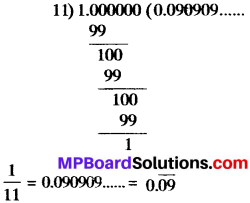The decimal expansion is non-terminating repeating.

3. 4$$\frac{1}{8}$$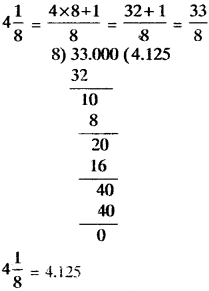The decimal expansion is terminating.

4. $$\frac{3}{13}$$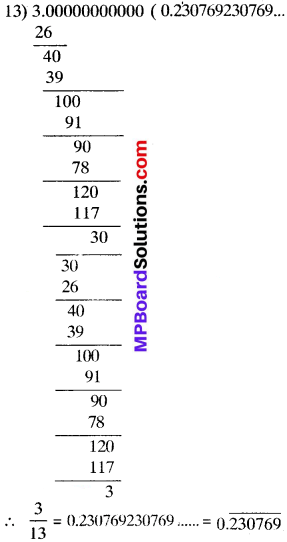The decimal expansion is non-terminating repeating.

5. $$\frac{2}{11}$$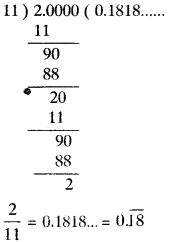The decimal expansion is non-terminating repeating.

6. $$\frac{329}{400}$$The decimal expansion is terminating.

Question 2.
You know that $$\frac{1}{7}$$ = $$\overline { 0.142857 }$$. Can vou predict what the decimal expansions of $$\frac{2}{7}$$, $$\frac{3}{7}$$, $$\frac{4}{7}$$, $$\frac{5}{7}$$, $$\frac{6}{7}$$ are, without actually doing the long division? If so, how?
[Hint: Study the remainders while finding the value of $$\frac{1}{7}$$ carefully.]
Solution:
$$\frac{1}{7}$$ = $$\overline { 0.142857 }$$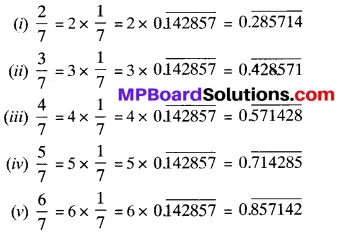Question 3.
Expressthe following in the form $$\frac{p}{q}$$, wherep and q are integers and q ≠ 0.

1. $$0 . \overline{6}$$
2. $$0 . \overline{oo1}$$

Solution:
1. $$0 . \overline{6}$$
Let x = $$0 . \overline{6}$$ …(i)
10x = $$6 . \overline{6}$$
[Multiplying (i) by 10 on both sides] …(ii)
Subtracting (i) from (ii). we get
9x = 6
x = $$\frac{6}{9}$$ = $$\frac{2}{3}$$
∴ $$6 . \overline{6}$$ = $$\frac{2}{3}$$

2. $$0 . \overline{oo1}$$
1000x = $$1 . \overline{001}$$
[Multiplying (i) by 1000] …(ii)
Subtracting (i) from (ii), we get
999x = 1
x = $$\frac{1}{999}$$
∴ $$0 . \overline{001}$$ = $$\frac{1}{999}$$

Question 4.
Express 0.99999….. in the form $$\frac{p}{q}$$. Are you surprised by vour answer? With your teacher and classmates, discuss why the answer make sense.
Solution:
0.99999 = $$0 . \overline{9}$$
Let x = 0.9 …(i)
10x = $$9 . \overline{9}$$
[Multiplying (i) by 10] …(ii)
Subtracting (i) from (ii), we get
9x = 9
x = $$\frac{9}{9}$$
∴ $$9 . \overline{9}$$ = 1

Question 5.
What can the maximum number of digits be in the repeating block of digits in the decimal expansion of $$\frac{1}{17}$$? Perform the division to check your answer.
Solution:
The maximum number of digits in the repeating block of digits in the decimal expansion $$\frac{1}{17}$$ can be 16.
0. 05882352941176470588235294117647….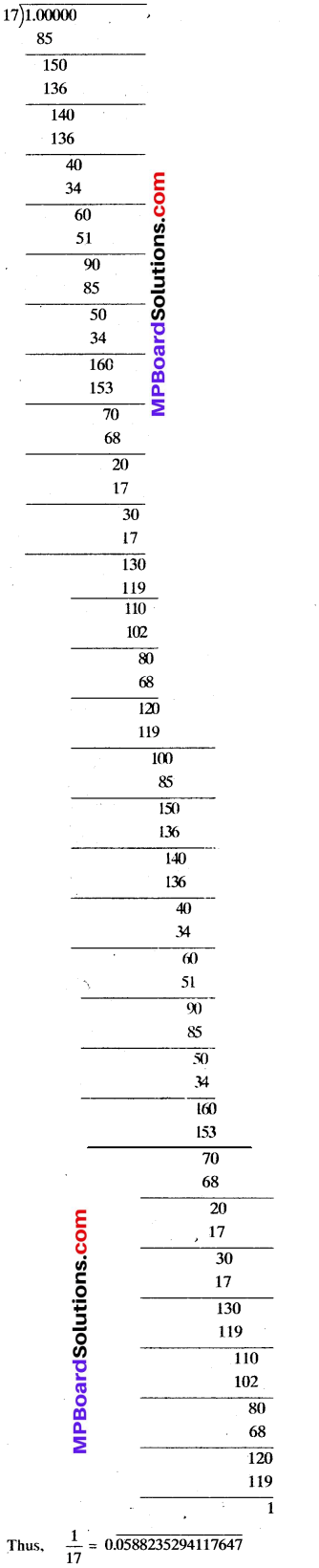By Long Division, the number of digits in the repeating block of digits in the decimal expansion of = $$\frac{1}{17}$$ = 16

Question 6.
Look at several examples of rational numbers in the form $$\frac{p}{q}$$ (q ≠ 0),where p and q are integers with no common factors other than 1 and having terminating decimal representation (expansions). Can you guess what property q must satisfy?
Solution:
Examples:The property that q must satisfy is that the prime factorisation of q have only powers of 2 or powers of 5 or both.

Question 7.
Write three numbers whose decimal expansions are non – terminating non – recurring.
Solution:
0. 01001000100001……..,
0. 20200220002200002…….,
0. 003000300003Question 8.
Find three different irrational numbers between the rational numbers $$\frac{5}{7}$$ and $$\frac{9}{11}$$.
Solution:
Irrational numbers between $$\frac{5}{7}$$ and $$\frac{9}{11}$$
$$\frac{5}{7}$$ = 0.71 and $$\frac{9}{11}$$ = 0.81
Three irrational numbers between $$\frac{5}{7}$$ and $$\frac{9}{11}$$ are
0. 7201001000…
0. 7301001000…
0. 7401001000…

Question 9.
Classify the following numbers as rational or irrational:

1. $$\sqrt{23}$$
2. $$\sqrt{225}$$
3. 0. 3796
4. 7. 478478…
5. 1. 101001000100001…

Solution:

1. $$\sqrt{23}$$ is an irrational number
2. $$\sqrt{225}$$ = 15, a rational number
3. 0. 3796 is a rational number
4. 7. 478478….. is an irrational number
5. 1. 101001000100001… is an irrational number